Courses

# Past Year Questions: Principal Stress and Principal Strain Notes | EduRev

## Civil Engineering (CE) : Past Year Questions: Principal Stress and Principal Strain Notes | EduRev

The document Past Year Questions: Principal Stress and Principal Strain Notes | EduRev is a part of the Civil Engineering (CE) Course Topic wise GATE Past Year Papers for Civil Engineering.
All you need of Civil Engineering (CE) at this link: Civil Engineering (CE)

Q.1 For a plane stress problem, the state of stress at a point P is represented by the stress element as shown in figure.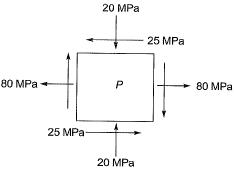By how much angle (θ) in degrees the stress element should be rotated in order to get the planes of maximum shear stress?    [2019 : 2 Marks, Set-II]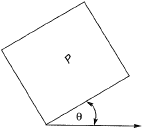(a) 31.7
(b) 13.3
(c) 26.6
(d) 48.3
Ans.
(A)
Solution: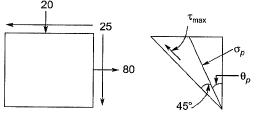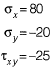Angle of plane of max shear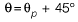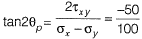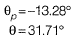Q.2 An element is subjected to biaxial normal tensile strains of 0.0030 and 0.0020. The normal strain in the plane of maximum shear strain is    [2019 : 1 Mark, Set-I]
(a) Zero
(b) 0.0050
(c) 0.0010
(d) 0.0025
Ans.
(D)
Solution: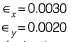Normal strain in the plane of maximum shear strain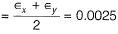Q.3 For the stress state (in MPa) shown in the figure, the major principal stress is 10 MPa.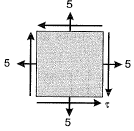The shear stress τ is    [2016 : 2 Marks, Set-II]
(a) 10.0 MPa
(b) 5.0 MPa
(c) 2.5 MPa
(d) 0.0 MPa
Ans.
(B)
Solution: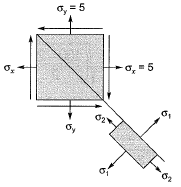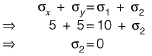Now,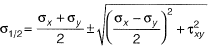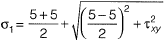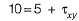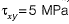Q.4 For the plane stress situation shown in the figure, the maximum shear stress and the plane on which it acts are    [2015 : 1 Mark, Set-II]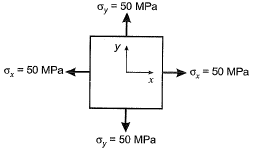(a) -50 MPa, on a plane 450 clockwise w.r.t. x-axis
(b) -50 MPa, on a plane 450 anti-clockwise w.r.t. x-axis
(c) 50 MPa, at all orientations
(d) Zero, at all orientations
Ans.
(D)
Solution:
Under hydrostatic loading condition, stresses at a point in all directions are equal and hence no shear stress.
Alternatively,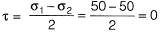Thus, Mohr’s circle reduces to a point. Hence shear stress at all orientations is zero.

Q.5 Two triangular wedges are glued together as shown in the following figure. The stress acting normal to the interface, σ is __________MPa.    [2015 : 1 Mark, Set-I]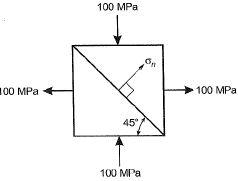Solution:

As plane ABand BCare principle planes, therefore Mohr’s circle for the given condition is,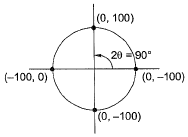Here, normal stress is zero at 450 to the principle plane.
Method-ll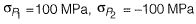⇒ at 450, it is a plane of maximum shear stress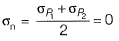Q.6 The state of 2D stress at a point is given by a matrix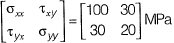The maximum shear stress in MPa is    [2013 : 2 Marks]
(a) 50
(b) 75
(c) 100
(d) 110
Ans.
(A)
Solution: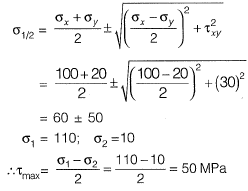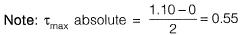But SS is not the option so taken (τmax)plain otherwise prefer (τmax)absolute.

Q.7 If a small concrete cube is submerged deep in still water in such a way that the pressure exerted on all faces of the cube is p, then the maximum shear stress developed inside the cube is   [2012 : 1 Mark]
(a) 0
(b) p/2
(c) p
(d) 2p
Ans.
(A)
Solution: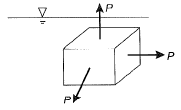Maximum shear stress,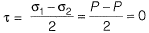Note: It is the case of hdyrostatic force, Mohr circle of which is a point hence τmax = 0.

Q.8 The major and minor principal stresses at a point are 3 MPa and - 3 MPa respectively. The maximum shear stress at the point is    [2010 : 1 Mark]
(a) zero
(b) 3 MPa
(c) 6 MPa
(d) 9 MP
Ans.
(B)
Solution: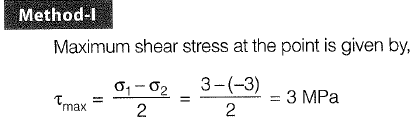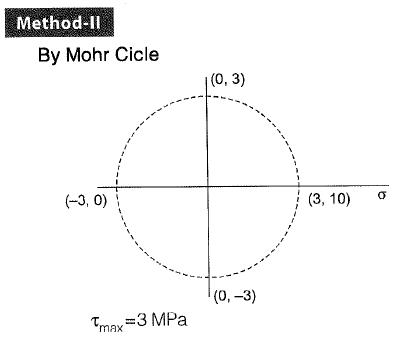Offer running on EduRev: Apply code STAYHOME200 to get INR 200 off on our premium plan EduRev Infinity!

69 docs

,

,

,

,

,

,

,

,

,

,

,

,

,

,

,

,

,

,

,

,

,

;